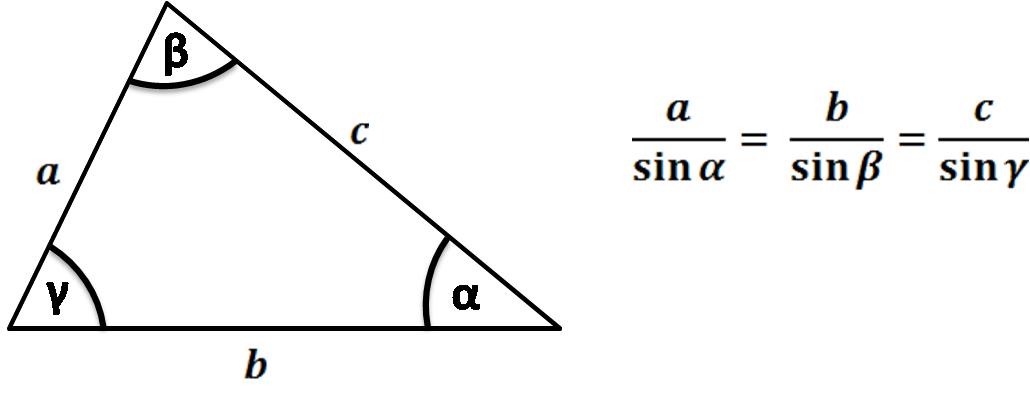# SSA Triangle Calculator

Created by Luis Fernando
Reviewed by Dominika Miszewska, MD, PhD candidate
Last updated: Jun 15, 2022

Welcome to our solve SSA triangle calculator, where you'll be able to solve SSA (side-side-angle) problems using the sine laws. This tool is also an ambiguous triangle calculator, as you can use it to solve the SSA ambiguity.

If you were searching for how to solve SSA triangles, you're in the right place! With this calculator, you can:

• Check if a triangle can exist;
• Check for SSA triangle congruence; and
• Know the ambiguous case of your triangle (if it applies).

## SSA triangle formula

This SSA triangle calculator uses the following formula:

$\frac{a}{\sin{\alpha}} = \frac{b}{\sin{\beta}} = \frac{c}{\sin{\gamma}}$

where the sides $a$, $b$, and $c$, and the angles $\alpha$, $\beta$, and $\gamma$ correspond to the shown in the following image:

The previous formulas correspond to the law of sines, and you can use them to solve SSA triangle problems.

## Example: How to solve an A = 46 a = 31 b = 27 triangle?

To solve a triangle of A = 46 a = 31 b = 27:

1. In the solve SSA triangle calculator, select a / sin(α) = b / sin(β) as the formula to use.
2. Input the following values in the calculator:
• Side a: 31;
• Side b: 27; and
• Angle α: 46°.
3. That's it! The unknown angle equals 38.794°. This SSA triangle has congruence and only one possible solution.

## FAQ

### Can SSA prove triangles are congruent?

No, an SSA triangle cannot prove a triangle is congruent. To check if an SSA triangle is congruent, you'll need a more detailed analysis or, even better, use our SSA triangle calculator.

### How do I solve an SSA triangle?

To solve an SSA triangle:

1. See if the info corresponds to two sides and an angle not in between them. If that's the case, you may have two possible answers.
2. Find the value of the unknown angle using sine law.
3. Subtract the previous angle from 180° to find the possible second angle.
4. Add the new angle to the originally known angle you were given at the beginning of the problem.
• If their sum is less than 180°, you have two valid answers.
• If their sum is higher than 180°, the second angle is not valid.
Luis Fernando
Which formula do you want to use?
a / sin(α) = b / sin(β)
Type 3 given values
Side a
in
Side b
in
Angle α
deg
Angle β
degPeople also viewed…

### Car vs. Bike

Everyone knows that biking is awesome, but only this Car vs. Bike Calculator turns biking hours into trees! 🌳

### Central angle

The central angle calculator finds the angle at the centre of a circle whose legs (radii) extend towards an arc along the circumference. Using two of the three parameters (central angle, radius, or arc length) find the third!

### Circle skirt

Circle skirt calculator makes sewing circle skirts a breeze.

### Multiplication

The multiplication calculator is here to find the product of your numbers.